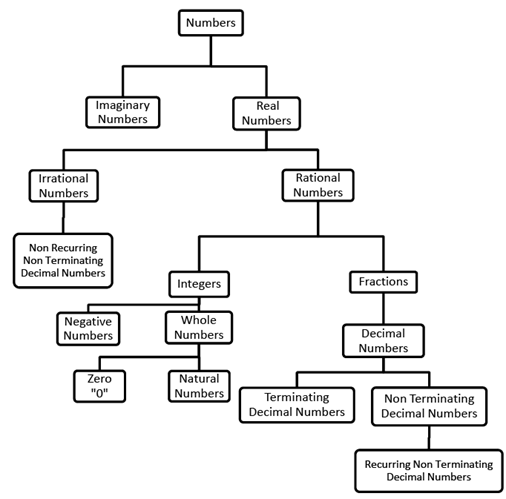Courses

# Number Systems: Overview with Examples- 2 Notes | EduRev

## CAT : Number Systems: Overview with Examples- 2 Notes | EduRev

The document Number Systems: Overview with Examples- 2 Notes | EduRev is a part of the CAT Course Quantitative Aptitude (Quant).
All you need of CAT at this link: CAT

1.4  THE NUMBER TREE1.5 ODD AND EVEN NUMBERS
This is a property of Integers. But since our questions are limited to the Whole numbers, we will keep our studies limited to them.
By Definition Even Numbers are those which are divisible by 2. They are the numbers with their unit digit as either 2, 4, 6, 8 or 0. The general expression of Even numbers is 2n where n is a whole number.
By Definition Odd Numbers are those which are not divisible by 2. They are the numbers with their unit digit as either 1, 3, 5, 7 or 9. The general expression of Odd numbers is 2n+1 where n is a whole number.

Certain Operations on Whole Numbers:
(i) Odd ± Odd = Even
(ii) Even ± Even = Even
(iii) Odd ± Even = Odd
(iv) Even ± Even ± Even ........... n terms = Even
(v) Odd ± Odd ± Odd .............. n terms = Even if n is Even, Odd if n is Odd
(vi) Odd × Odd = Odd
(vii) Even × Odd = Even
(viii) Even × Even = Even
(ix) Even × Even × Even ....... n terms = Even
(x) Odd × Odd × Odd ....... n terms = Odd
(xi) (Even)n = Even
(xii) (odd)n = Odd

Question 1:If a is even what would 5a + 1 be

Question 2:If a is even and b is odd what would a3 + 3b + 6 be

Question 3:If 5a - 2 is even what would a  be

1.6 PRIME AND COMPOSITE NUMBERS
Being Prime is a property of Natural Numbers. All those numbers which are divisible by exactly 2 numbers i.e. itself and unity "1".
Example: 2, 3, 5, 7, 11 etc.

Composite are the numbers which are divisible by more than 2 numbers i.e. itself, unity "1" and at least one other.
Example: 4, 6, 8, 9, 10 etc.

1 is neither Prime nor Composite.

Question 4:How many Prime numbers are there less than 100

Question 5:Which of the following cannot be the sum of two prime numbers.

Question 6:State Whether True or False

All Prime numbers are Odd

Question 7:State Whether True or False

Sum of Any 2 prime numbers is always Even

Question 8:State Whether True or False

Product of Any two prime numbers is always Odd

Question 9:State Whether True or False

There are no 4 digit Prime numbers.

Property of Prime Numbers:
All Prime numbers greater than 3 are of the form 6n ± 1 but not all numbers of the form 6n ± 1 are Prime. This property means when prime numbers greater than 3 are divided by 6 they leave a remainder of 1 or 5.

Question 10:Which of the following is a prime numbers.

1.7 CO - PRIME NUMBERS
This is a property of a pair or more than two natural numbers. Two numbers are said to be co-prime if the only number which can completely divide both the numbers is 1. No number other than 1 can divide both the given numbers of the pair. These numbers in themselves can be prime or composite. 1 is co-prime with all the natural numbers other than 1.
Example: (3, 7); (4, 15); (3, 10) etc.

A set of numbers is said to be co-prime if their HCF is 1. We will be learning about HCF in the following chapters.
Example: (3, 7, 11); (4, 15, 10); (3, 10, 8) etc.

Offer running on EduRev: Apply code STAYHOME200 to get INR 200 off on our premium plan EduRev Infinity!

,

,

,

,

,

,

,

,

,

,

,

,

,

,

,

,

,

,

,

,

,

;